# Texas Go Math Grade 4 Lesson 5.1 Answer Key Add and subtract parts of a whole

Refer to our Texas Go Math Grade 4 Answer Key Pdf to score good marks in the exams. Test yourself by practicing the problems from Texas Go Math Grade 4 Lesson 5.1 Answer Key Add and subtract parts of a whole.

## Texas Go Math Grade 4 Lesson 5.1 Answer Key Add and subtract parts of a whole

Essential Question

When con you odd or subtract ports of o whole?
Explanation:
When the denominators are same

Materials

• fraction circles
• color pencils

Ms. Clark has the following pie pieces left over from a bake sale.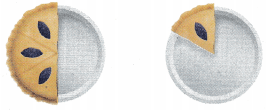She will combine the pieces so they are on the same dish. How much pie will be on the dish?
Answer: $$\frac{2}{3}$$
Explanation:
$$\frac{4}{6}$$  that is $$\frac{2}{3}$$ pie will be on the dish

A. Model the problem using fraction circles. Draw a picture of your model. Then write the sum.So, ___________ of a pie is on the dish.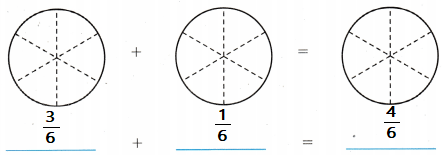So, $$\frac{4}{6}$$ of a pie is on the dish.

B. Suppose Ms. Clark eats 2 pieces of the pie. How much pie will be left on the dish? Model the problem using fraction circles. Draw a picture of your model. Then write the difference.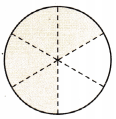______________ – ____________ = ____________
So, _____________ of the pie is left on the dish.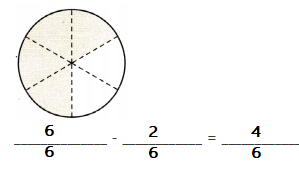Explanation:
So, $$\frac{4}{6}$$ of the pie is left on the dish.

Make connections

You can only join or separate parts that refer to the same whole.
Suppose Randy has $$\frac{1}{4}$$ of a round cake and $$\frac{1}{4}$$ of a square cake.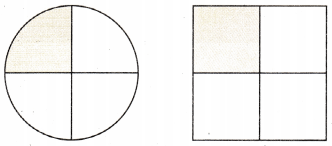a. Are the wholes the same? Explain.
Explanation:
Both the shapes are different.
The wholes are not same.

b. Does the sum $$\frac{1}{4}+\frac{1}{4}=\frac{2}{4}$$ make sense in this situation? Explain.
Explanation:
Both the shapes are different
The sum $$\frac{1}{4}+\frac{1}{4}=\frac{2}{4}$$ make sense in this situation

Math Talk

Mathematical Processes
Give an example of a situation where the equation $$\frac{1}{4}+\frac{1}{4}=\frac{2}{4}$$ makes sense. Explain yourExplanation:
The equation $$\frac{1}{4}+\frac{1}{4}=\frac{2}{4}$$ makes sense in this situation.
The whole is same when the shapes are same

Share and Grow

Use the model to write an equation.

Question 1.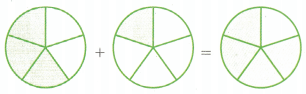Explanation:
$$\frac{3}{5}$$ + $$\frac{1}{5}$$ = $$\frac{4}{5}$$

Question 2.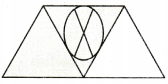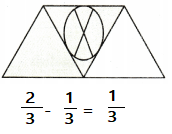Explanation:
A trapezoid is divided into 3 triangles

Question 3.
Multi-Step Sean has $$\frac{1}{5}$$ of a cupcake and $$\frac{1}{5}$$ of a large cake.
a. Are the wholes the same? Explain.
Explanation:
The size cup cake and large cake are different.

b. Does the sum $$\frac{1}{5}+\frac{1}{5}$$ = $$\frac{2}{5}$$ make sense in this situation? Explain.
Explanation:
Equation is correct but not in this situation.

Use the model to solve the equation.

Question 4.
$$\frac{3}{4}-\frac{1}{4}$$ = _____________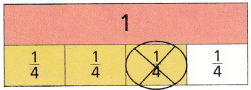Answer: $$\frac{2}{4}$$
Explanation:
According to this equation $$\frac{3}{4}-\frac{1}{4}$$  simplest form is $$\frac{2}{4}$$

Question 5.
$$\frac{5}{6}+\frac{1}{6}$$ = _____________$$\frac{5}{6}+\frac{1}{6}$$ = 1
Explanation:
When you divide a number with the same number the answer is 1.

Problem Solving

H.O.T. Sense or Nonsense?

Question 6.
Samantha and Kim used different models to help find $$\frac{1}{3}+\frac{1}{3}$$ Whose model makes sense? Whose Model is nonsense? Explain your reasoning below each model.Explanation:
Kim’s model make sense.

Question 7.
H.O.T. Justify If there is $$\frac{4}{6}$$ of a pie on a plate. what part of the pie is missing from the plate? Write an equation to justify your answer.
$$\frac{4}{6}$$ + $$\frac{2}{6}$$ = 1
Explanation:
$$\frac{2}{6}$$ of the pie is missing from the plate

Use models to solve.

Question 8.
At lunch yesterday, Ryan ate $$\frac{2}{6}$$ of an apple and I ate $$\frac{2}{6}$$ of the apple. Together, how much of the apple did we eat?
(A) $$\frac{2}{6}$$
(B) $$\frac{4}{6}$$
(C) $$\frac{2}{12}$$
(D) $$\frac{4}{12}$$
Explanation:
$$\frac{2}{6}$$ + $$\frac{2}{6}$$ = $$\frac{4}{6}$$
$$\frac{4}{6}$$ they ate the apple.

Question 9.
At the start of art class, Logan had $$\frac{7}{12}$$ of a block of clay. After class, $$\frac{5}{12}$$ of the block was left. What fraction of the block did Logan use during class?
(A) $$\frac{7}{12}$$
(B) $$\frac{12}{12}$$
(C) $$\frac{5}{12}$$
(D) $$\frac{2}{12}$$
Explanation:
$$\frac{7}{12}$$ – $$\frac{5}{12}$$ = $$\frac{2}{12}$$
Used the simplest form

Question 10.
Multi-Step Samantha is mixing batter for muffins. She mixes $$\frac{2}{4}$$ cup of flour and $$\frac{1}{4}$$ cup of sugar, Then she adds $$\frac{1}{4}$$ cup of milk. How much muffin batter has she mixed so far?
(A) $$\frac{3}{8}$$ cup
(B) $$\frac{2}{4}$$ cup
(C) 1 cup
(D) $$\frac{3}{4}$$ cup
Explanation:
$$\frac{2}{4}$$ + $$\frac{1}{4}$$  + $$\frac{1}{4}$$ = 1
Used the simplest form

TEXAS Test Prep

Question 11.
Which equation matches the model?(A) $$\frac{1}{4}+\frac{3}{4}=\frac{4}{4}$$
(B) $$\frac{1}{4}+\frac{2}{4}=\frac{3}{4}$$
(C) $$\frac{3}{4}+\frac{2}{4}=\frac{5}{4}$$
(D) $$\frac{1}{8}+\frac{2}{8}=\frac{3}{8}$$
Explanation:
$$\frac{1}{4}+\frac{2}{4}=\frac{3}{4}$$ This equation matches the model

### Texas Go Math Grade 4 Lesson 5.1 Homework and Practice Answer Key

Use the model to write an equation.

Question 1.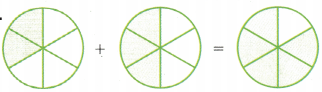$$\frac{2}{6}$$ + $$\frac{3}{6}$$  = $$\frac{5}{6}$$
Explanation:
Written equation for above model.

Question 2.$$\frac{3}{4}$$ – $$\frac{1}{4}$$  = $$\frac{2}{4}$$
Explanation:
The above parallelogram is divided into 4 equal parts in that 3 are shaded
in that 1 is filled with image

Use the model to solve an equation.

Question 3.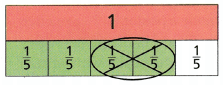Answer: $$\frac{2}{5}$$
Explanation:
$$\frac{4}{5}$$ – $$\frac{2}{5}$$  = $$\frac{2}{5}$$
By using the model solved the equation.

Question 4.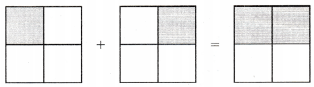$$\frac{1}{4}$$ + $$\frac{1}{4}$$ = $$\frac{2}{4}$$
Explanation:
By using the model solved the equation.

Question 5.
If there is $$\frac{6}{8}$$ of a pizza on a plate, what part of the pizza is missing from the plate? Write an equation to justify your answer.
Answer: $$\frac{2}{8}$$
Explanation:
1 – $$\frac{6}{8}$$ =  $$\frac{2}{8}$$
$$\frac{2}{8}$$  part of the pizza is missing from the plate.

Problem Solving

Question 6.
If there is $$\frac{3}{8}$$ of a pizza on a plate? what part of the pizza is missing from the plate? Write an equation to justify your answer.
Answer: $$\frac{5}{8}$$
Explanation:
1 – $$\frac{3}{8}$$ =  $$\frac{5}{8}$$
$$\frac{5}{8}$$  part of the pizza is missing from the plate.

Question 7.
Maria is making cupcakes. She fills $$\frac{4}{12}$$ of the cups with chocolate batter and $$\frac{7}{12}$$ of the cups with vanilla batter. How many of the cups has Maria filled? Write an equation to justify your answer.
Answer: $$\frac{11}{12}$$
Explanation:
$$\frac{4}{12}$$ + $$\frac{7}{12}$$  = $$\frac{11}{12}$$
Maria filled $$\frac{11}{12}$$  cups.

Lesson Check

Question 8.
which equation matches the model?(A) $$\frac{2}{8}+\frac{1}{8}=\frac{3}{8}$$
(B) $$\frac{2}{4}+\frac{3}{4}=\frac{5}{4}$$
(C) $$\frac{2}{4}+\frac{1}{4}=\frac{3}{4}$$
(D) $$\frac{1}{4}+\frac{3}{4}=\frac{4}{4}$$
Explanation:
$$\frac{2}{4}+\frac{1}{4}=\frac{3}{4}$$  equation matches the model

Question 9.
Which equation matches the model?(A) $$\frac{5}{7}-\frac{2}{7}=\frac{3}{7}$$
(B) $$\frac{7}{7}-\frac{5}{7}=\frac{2}{7}$$
(C) $$\frac{7}{7}-\frac{2}{7}=\frac{5}{7}$$
(D) $$\frac{5}{7}-\frac{3}{7}=\frac{2}{7}$$
Explanation:
$$\frac{5}{7}-\frac{2}{7}=\frac{3}{7}$$ equation matches the model

Question 10.
In Dylan’s family $$\frac{5}{6}$$ of the children have brown hair. The rest of the children have blond hair.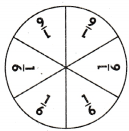What fraction of the children has blond hair?
(A) $$\frac{4}{6}$$
(B) $$\frac{2}{6}$$
(C) $$\frac{3}{6}$$
(D) $$\frac{1}{6}$$
Explanation:
1 – $$\frac{5}{6}$$  = $$\frac{1}{6}$$
$$\frac{1}{6}$$ fraction of the children has blond hair

Question 11.
Miranda made a poster for her science project. She filled $$\frac{3}{8}$$ of the poster with photos and $$\frac{4}{8}$$ of the poster with written information. how much space has she filled on lier poster so far?
(A) $$\frac{7}{8}$$
(B) $$\frac{1}{8}$$
(C) $$\frac{6}{8}$$
(D) $$\frac{2}{8}$$
Explanation:
$$\frac{3}{8}$$ +  $$\frac{4}{8}$$  = $$\frac{7}{8}$$
$$\frac{1}{8}$$ space has she filled on lier poster so far

Question 12.
Multi-Step Carrie’s dance class learned $$\frac{1}{5}$$ of a new dance on Monday, and $$\frac{2}{5}$$ of the dance on Tuesday. What fraction of the dance is left for the class to learn on Wednesday?
(A) $$\frac{4}{5}$$
(B) $$\frac{3}{5}$$
(C) $$\frac{2}{5}$$
(D) $$\frac{1}{5}$$
Explanation:
$$\frac{1}{5}$$ + $$\frac{2}{5}$$ = $$\frac{3}{5}$$
1 – $$\frac{3}{5}$$ = $$\frac{1}{5}$$
$$\frac{1}{5}$$ fraction of the dance is left for the class to learn on Wednesday

Question 13.
Multi-Step Mrs. Simon planted $$\frac{4}{12}$$ of her flowers in her front yard, $$\frac{3}{12}$$ of her flowers in her back yard, and $$\frac{2}{12}$$ of her flowers on the side of her house. What fraction of her flowers has she planted so far?
(A) $$\frac{2}{12}$$
(B) $$\frac{9}{12}$$
(C) $$\frac{3}{12}$$
(D) $$\frac{10}{12}$$
$$\frac{4}{12}$$ + $$\frac{3}{12}$$ + $$\frac{2}{12}$$ = $$\frac{9}{12}$$